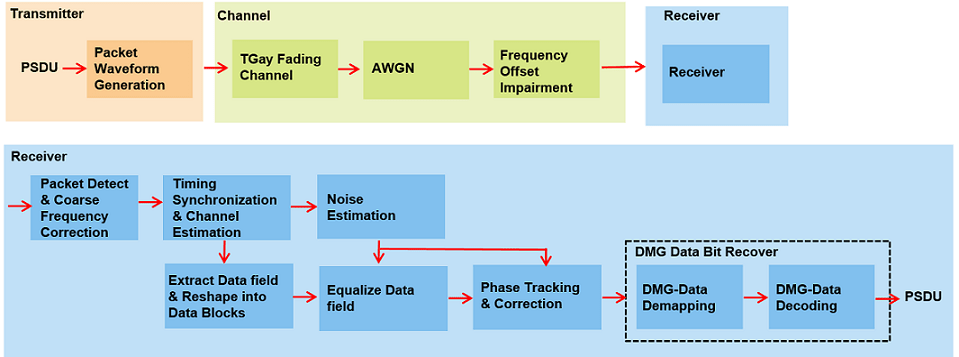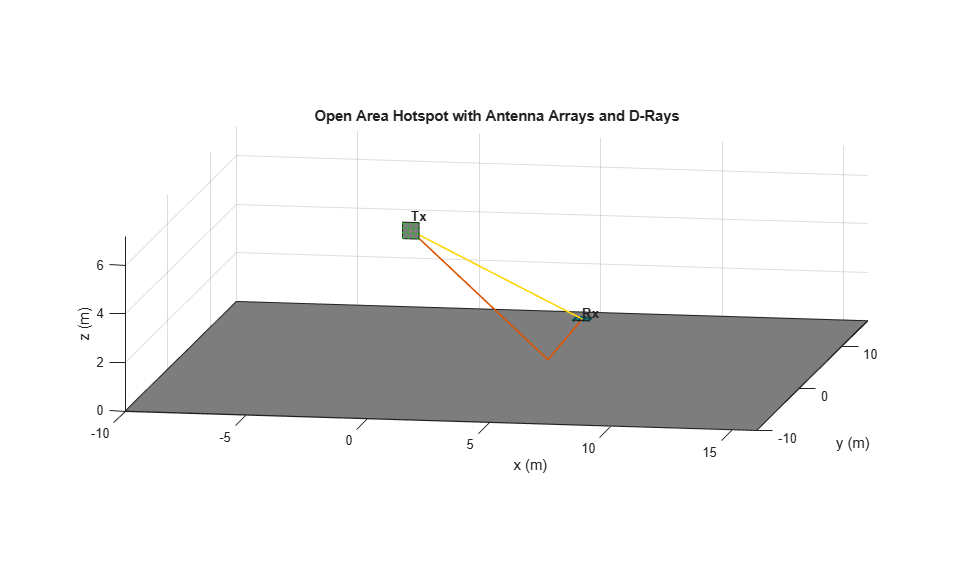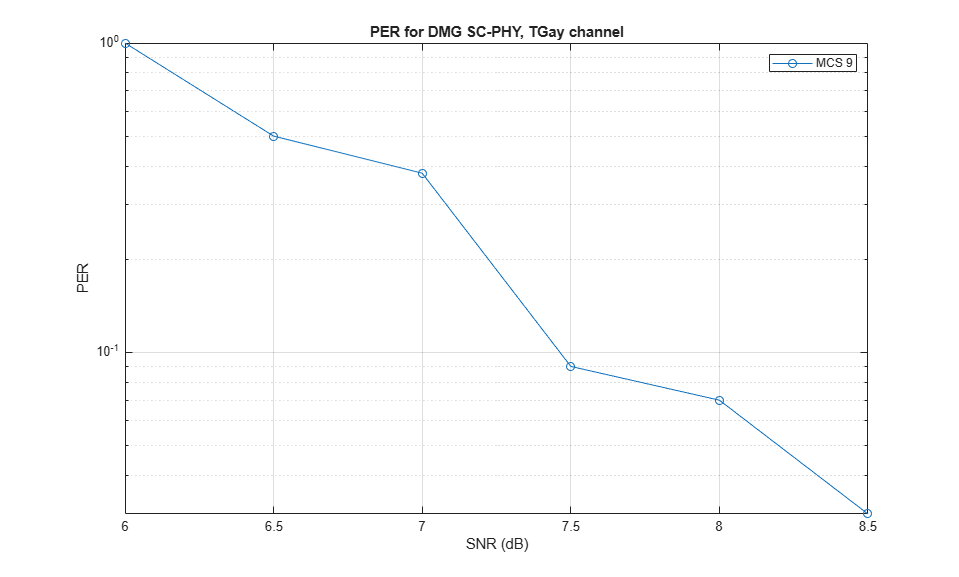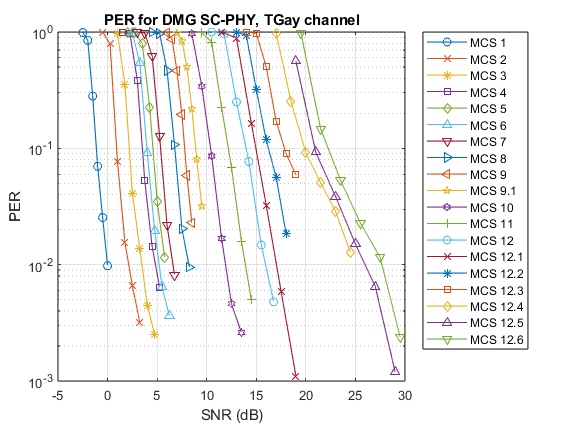## 802.11ad Packet Error Rate Single Carrier PHY Simulation with TGay Channel

This example shows how to measure the packet error rate of an IEEE® 802.11ad™ DMG single carrier (SC) PHY link using an end-to-end simulation.

### Introduction

In this example an end-to-end simulation is used to determine the packet error rate for an 802.11ad SC [ 1 ] link with a TGay millimeter-wave channel [ 2 ] at a selection of SNR points. At each SNR point multiple packets are transmitted through the fading channel, synchronized, demodulated and the PSDUs recovered. Carrier frequency offset and a time delay are also modeled. The PSDUs are compared to those transmitted to determine the number of packet errors and hence the packet error rate. The processing for each packet is summarized in the following diagram.This example also demonstrates how a `parfor` loop can be used instead of the `for` loop when simulating each SNR point to speed up a simulation. `parfor`, as part of the Parallel Computing Toolbox™, executes processing for each SNR in parallel to reduce the total simulation time.

### Waveform Configuration

An 802.11ad DMG SC PHY transmission is simulated in this example. The DMG format configuration object contains the format specific configuration of the transmission. The object is created using the `wlanDMGConfig` function. The properties of the object contain the configuration of the transmitted packet. In this example the object is configured to generate a single carrier waveform of MCS "9". The MCS determines the PHY type used and in this example it must be a string within the range 1-12, or one of {9.1 12.1 12.2 12.3 12.4 12.5 12.6} to simulate the SC PHY.

```% Create a format configuration object cfgDMG = wlanDMGConfig; mcs = "9"; % MCS specified as a string scalar or string vector cfgDMG.PSDULength = 4096; % PSDULength in bytes ```

In this example we simulate a TGay channel model for the open area hotspot scenario, using the `wlanTGayChannel` object. Both the transmit and receive arrays are a 4x4 uniform rectangular array (URA). Ray-tracing is performed from the transmit to the receive array to derive two deterministic rays: one LOS ray and another NLOS ray with one-order reflection from the ground. Random rays and intra-cluster rays are subsequently generated according to the quasi-deterministic (Q-D) modeling approach and parameters in [ 2 ]. Beamforming is performed to set the transmit and receive antenna steering direction along the ray with the maximum power. The fading channel impulse responses are normalized so no power gain or loss is introduced by the channel. Both the channel input and output signals are unpolarized.

```% Get sampling rate and specify carrier frequency fs = wlanSampleRate(cfgDMG); fc = 60e9; % Create a TGay channel object tgayChan = wlanTGayChannel; tgayChan.SampleRate = fs; tgayChan.CarrierFrequency = fc; tgayChan.Environment = 'Open area hotspot'; tgayChan.TransmitArray.Size = [4 4]; tgayChan.TransmitArrayPosition = [0; 0; 6]; % Meters tgayChan.TransmitArrayOrientation = [0; 270; 0]; % Degrees tgayChan.ReceiveArray.Size = [4 4]; tgayChan.ReceiveArrayPosition = [6; 6; 1.5]; % Meters tgayChan.ReceiveArrayOrientation = [90; 0; 0]; % Degrees tgayChan.BeamformingMethod = 'Maximum power ray'; tgayChan.NormalizeImpulseResponses = true; ```

Given the channel object configuration, we display a 3D map to show the environment and antenna array settings. The two deterministic rays from ray-tracing are also shown in the figure.

```showEnvironment(tgayChan); ```### Channel Impairment

The maximum tolerance for the transmitter center frequency must be within [-20, +20] ppm [ 1 ]. In this example, a clock accuracy of 20ppm is considered to derive the CFO. The transmitted signal is delayed by 500 samples and also appended by 100 zero samples at the end to account for delays from TGay channel filtering.

```ppm = 20; % Clock accuracy to drive the CFO (ppm) freqOffset = ppm*1e-6*fc; % Carrier frequency offset (Hz) delay = 500; % Sample to delay the waveform zeroPadding = 100; % Add trailing zeros to allow for channel delay ```

### Simulation Parameters

For each SNR point (dB) in the cell `snrRanges` a number of packets are generated, passed through a channel and demodulated to determine the packet error rate. The SNR points to test are selected from `snrRanges` based on the MCS simulated.

```snrRanges = {-2.0:0.5:0.5, ... % MCS 1 0.0:1.0:5.0, ... % MCS 2 1.0:1.2:7.0, ... % MCS 3 2.0:1.2:8.0, ... % MCS 4 2.0:1.3:8.5, ... % MCS 5 2.5:1.3:9.0, ... % MCS 6 4.0:1.3:10.5, ... % MCS 7 5.0:1.5:12.5,... % MCS 8 5.5:1.5:13.0, ... % MCS 9 7.0:1.5:14.5, ... % MCS 9.1 8.0:1.8:17.0, ... % MCS 10 10.0:2.0:20.0, ... % MCS 11 12.0:2.0:22.0, ... % MCS 12 12.0:2.0:22.0, ... % MCS 12.1 14.0:2.0:24.0, ... % MCS 12.2 16.0:2.5:28.5, ... % MCS 12.3 17.0:2.5:29.5, ... % MCS 12.4 17.0:2.5:29.5, ... % MCS 12.5 20.0:2.5:32.5}; % MCS 12.6 ```

The number of packets tested at each SNR point is controlled by two parameters:

1. `maxNumErrors` is the maximum number of packet errors simulated at each SNR point. When the number of packet errors reaches this limit, the simulation at this SNR point is complete.

2. `maxNumPackets` is the maximum number of packets simulated at each SNR point and limits the length of the simulation if the packet error limit is not reached.

The numbers chosen in this example will lead to a very short simulation. For meaningful results we recommend increasing the numbers.

```maxNumErrors = 10; % The maximum number of packet errors at an SNR point maxNumPackets = 100; % Maximum number of packets at an SNR point ```

### Processing SNR Points

For each SNR point a number of packets are tested and the packet error rate calculated.

For each packet the following processing steps occur:

1. A PSDU is created and encoded to create a single packet waveform.

2. The waveform is passed through a TGay channel model. Different channel realizations are modeled for different packets.

3. AWGN is added to the received waveform. `comm.AWGNChannel` is configured to provide the correct SNR.

4. The frequency offset impairment is added to each packet.

5. The packet is detected.

6. Carrier frequency offset is estimated and corrected.

7. Fine timing synchronization is established. The CE field samples are provided for fine timing to allow for packet detection at the start of the STF.

8. The STF and CE fields are extracted from the synchronized received waveform. The noise and channel estimation is performed on the recovered fields respectively.

9. The data field, excluding the first guard interval is extracted and reshaped into blocks. The received symbols in the data field are equalized.

10. The received symbols are tracked and corrected for phase errors caused by any residual carrier frequency offset.

11. The data field is decoded to recover the PSDU bits.

A `parfor` loop can be used to parallelize processing of the SNR points, therefore for each SNR point an AWGN channel is created and configured with `comm.AWGNChannel`. To enable the use of parallel computing for increased speed comment out the `for` statement and uncomment the `parfor` statement below.

```numSNR = numel(snrRanges{1}); % Number of SNR points if ~isstring(mcs) error('MCS must be specified as a string scalar or string vector'); end numMCS = numel(mcs); % Number of MCS packetErrorRate = zeros(numMCS,numSNR); Ngi = 64; % Fixed GI length defined in the standard (20.6.3.2.5) validMCS = string(sort([1:12 9.1 12.1:0.1:12.6])); for imcs = 1:numMCS cfgDMG.MCS = mcs(imcs); if ~strcmp(phyType(cfgDMG),'SC') error('This example only supports DMG SC PHY simulation'); end ind = wlanFieldIndices(cfgDMG); snr = snrRanges{mcs(imcs)==validMCS}; % SNR points to simulate from MCS % parfor isnr = 1:numSNR % Use 'parfor' to speed up the simulation for isnr = 1:numSNR % Use 'for' to debug the simulation % Set random substream index per iteration to ensure that each % iteration uses a repeatable set of random numbers stream = RandStream('combRecursive','Seed',10); stream.Substream = isnr; RandStream.setGlobalStream(stream); % Create an instance of the AWGN channel per SNR point simulated awgnChannel = comm.AWGNChannel; awgnChannel.NoiseMethod = 'Signal to noise ratio (SNR)'; awgnChannel.SNR = snr(isnr); % Set simulation parameters numPacketErrors = 0; numPkt = 1; % Index of the transmitted packet while numPacketErrors<=maxNumErrors && numPkt<=maxNumPackets % Generate a packet waveform psdu = randi([0 1],cfgDMG.PSDULength*8,1); txWaveform = wlanWaveformGenerator(psdu,cfgDMG); % Add delay and trailing zeros tx = [zeros(delay,1); txWaveform; zeros(zeroPadding,1)]; % Transmit through a TGay channel. Reset the channel for a % different realization per packet. reset(tgayChan); chanOut = tgayChan(tx); % Add noise rx = awgnChannel(chanOut); % Add CFO rx = helperFrequencyOffset(rx,fs,freqOffset); % Packet detection threshold = 0.03; % Good for low SNRs pktStartOffset = dmgPacketDetect(rx,0,threshold); if isempty(pktStartOffset) % If empty no STF detected; packet error numPacketErrors = numPacketErrors+1; numPkt = numPkt+1; continue; % Go to next loop iteration end % Frequency offset estimation and correction stf = rx(pktStartOffset+(ind.DMGSTF(1):ind.DMGSTF(2))); fOffsetEst = dmgCFOEstimate(stf); rx = helperFrequencyOffset(rx,fs,-fOffsetEst); % Symbol timing and channel estimate preamblefield = rx(pktStartOffset+1:pktStartOffset+ind.DMGHeader(2),:); [symbolTimingOffset,chanEst] = dmgTimingAndChannelEstimate(preamblefield); startOffset = pktStartOffset+symbolTimingOffset; % If not enough samples to decode detected data field start, % then assume synchronization error and packet error if (startOffset+ind.DMGData(2))>size(rx,1) numPacketErrors = numPacketErrors+1; numPkt = numPkt+1; continue; % Go to next loop iteration end % Noise estimation using the STF as repeating sequence stf = rx(pktStartOffset+(ind.DMGSTF(1):ind.DMGSTF(2))); nVarEst = dmgSTFNoiseEstimate(stf); % Extract data field (ignore first GI) rxData = rx(startOffset+((ind.DMGData(1)+Ngi):ind.DMGData(2))); % Linear frequency domain equalization rxEqDataBlks = dmgSingleCarrierFDE(rxData,chanEst,nVarEst); % Unique word phase tracking rxEqDataBlks = dmgUniqueWordPhaseTracking(rxEqDataBlks); % Discard GI from all blocks rxDataSym = rxEqDataBlks(1:end-Ngi,:); % Recover the transmitted PSDU from DMG Data field dataDecode = wlanDMGDataBitRecover(rxDataSym,nVarEst,cfgDMG); % Determine if any bits are in error, i.e. a packet error packetError = any(biterr(psdu,dataDecode)); numPacketErrors = numPacketErrors+packetError; numPkt = numPkt+1; end % Calculate packet error rate (PER) at SNR point packetErrorRate(imcs,isnr) = numPacketErrors/(numPkt-1); disp(join([" MCS:" cfgDMG.MCS ", SNR " ... num2str(snr(isnr)) " completed after " ... num2str(numPkt-1) " packets, PER: " ... num2str(packetErrorRate(imcs,isnr))],"")); end end ```
``` MCS:9, SNR 5.5 completed after 11 packets, PER: 1 MCS:9, SNR 7 completed after 14 packets, PER: 0.78571 MCS:9, SNR 8.5 completed after 20 packets, PER: 0.55 MCS:9, SNR 10 completed after 35 packets, PER: 0.31429 MCS:9, SNR 11.5 completed after 70 packets, PER: 0.15714 MCS:9, SNR 13 completed after 100 packets, PER: 0.08 ```

### Plot Packet Error Rate vs SNR Results

```markers = 'ox*sd^v><ph+ox*sd^v'; color = 'bmcrgbrkymcrgbrkymc'; figure; for imcs = 1:numMCS semilogy(snrRanges{mcs(imcs)==validMCS},packetErrorRate(imcs,:).',['-' markers(imcs) color(imcs)]); hold on; end grid on; xlabel('SNR (dB)'); ylabel('PER'); dataStr = arrayfun(@(x)sprintf('MCS %s',x),mcs,'UniformOutput',false); legend(dataStr); title('PER for DMG SC-PHY, TGay channel'); ```### Further Exploration

The number of packets tested at each SNR point is controlled by two parameters; `maxNumErrors` and `maxNumPackets`. For meaningful results, it is recommended that these values should be larger than those presented in this example. Increasing the number of packets simulated allows the PER under different scenarios to be compared. Try changing the MCS value and compare the packet error rate. As an example, the figure below was created by running the example for all single carrier MCS with `PSDULength`: 8192 bytes, `maxNumErrors`: 1000 and `maxNumPackets`: 10000.Explore the TGay channel settings by changing the environment ('Street canyon hotspot' or 'Large hotel lobby'), user configurations, polarization type, array configurations, beamforming method and so on. In the street canyon scenario, the object calculates deterministic rays up to 1-order reflection from the ground and walls. In the hotel lobby scenario, the deterministic rays are up to 2-order reflection from the ground, ceiling and/or walls. Due to the additional reflection of rays, the PER results are typically better than the above obtained from the open area scenario.

### Appendix

This example uses the following helper functions and objects:

### Selected Bibliography

1. IEEE Std 802.11ad™-2012 IEEE Standard for Information technology - Telecommunications and information exchange between systems - Local and metropolitan area networks - Specific requirements - Part 11: Wireless LAN Medium Access Control (MAC) and Physical Layer (PHY) Specifications. Amendment 3: Enhancements for Very High Throughput in the 60 GHz Band.

2. A. Maltsev and et. al, Channel Models for IEEE 802.11ay, IEEE 802.11-15/1150r9, Mar. 2017.

﻿
##### Support